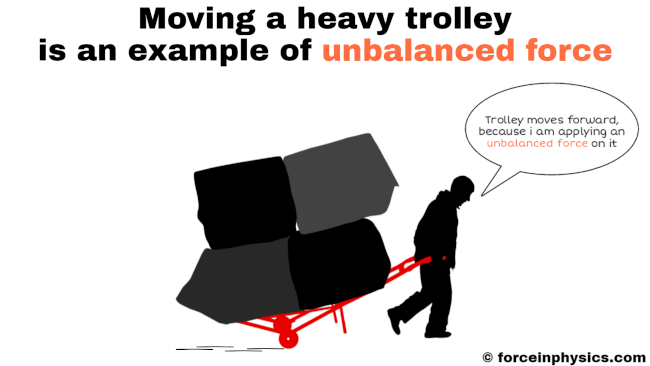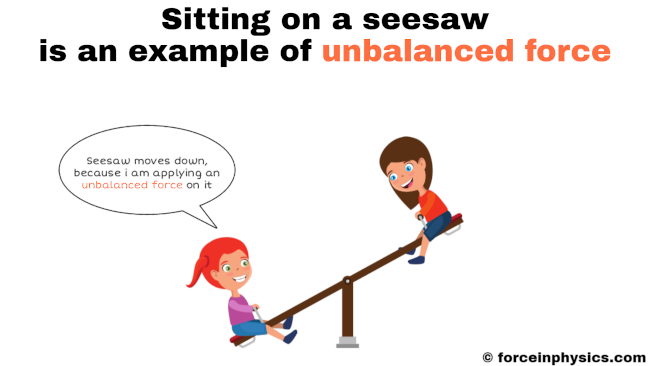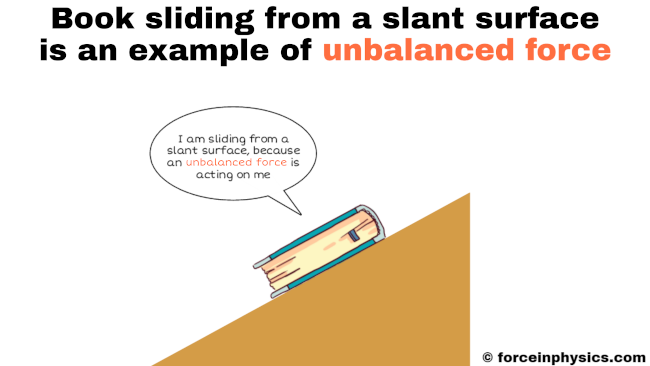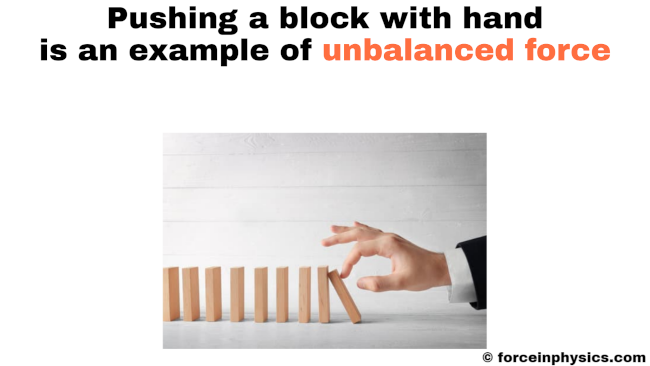# Unbalanced force

An unbalanced force, often encountered in everyday scenarios, is a force that leads to a change in an object’s motion. Unlike balanced forces, unbalanced forces do not offset or cancel each other out. Instead, they create a net force that acts on the object, causing it to accelerate or decelerate. For instance, when a person pushes a table lying on the floor, the force they apply is unbalanced as it exceeds the opposing forces like friction. Consequently, the table begins to move in the direction of the applied force. In this way, unbalanced forces disrupt the equilibrium of an object and produce a noticeable effect on its motion.

Contents

## Examples

### Football

When an individual kicks a football, the force exerted by their leg on the ball acts as an unbalanced force, initiating the ball’s motion. The force of the kick overcomes the ball’s initial state of rest, propelling it forward in the direction of the kick. As a result of this unbalanced force, the football starts moving, illustrating the concept of unbalanced forces causing a change in the object’s motion.

### TrolleyThe force exerted to move the heavy luggage trolley is unbalanced, causing it to accelerate in the direction of the applied force

When a person pulls a heavy luggage trolley, an unbalanced force is at play. Unbalanced forces arise when the net force acting on an object is non-zero, leading to changes in motion. As the person exerts force on the trolley to move it, this applied force becomes unbalanced, resulting in acceleration. The trolley will accelerate if the force applied by the person is greater than the opposing forces, such as friction and air resistance. On the other hand, if the opposing forces outweigh the applied force, the trolley will decelerate or come to a stop. This example illustrates how unbalanced forces cause objects to change their motion when external forces are not in equilibrium.

### SeesawIf one child is heavier than the other, the seesaw will tilt due to an unbalanced force, with the heavier child’s side moving downward

A seesaw perfectly illustrates the concept of unbalanced force. When two individuals of different weights sit on each end, an imbalance of forces occurs. The side with the heavier person exerts a stronger downward force, causing that end to lower while the other end rises. This unequal distribution of forces results in an unbalanced force, leading to the dynamic motion of the seesaw. The seesaw continues to tilt until the forces become balanced or until an external force comes into play. This example demonstrates how unbalanced forces cause objects to move when opposing forces are not in equilibrium, creating an exciting playtime experience on the seesaw.

### Sliding bookThe decrease in the normal force on the book creates an unbalanced force, allowing it to slide down the slanted surface due to the force of gravity

When a book is placed on a slanted surface, it experiences an unbalanced force that initiates the sliding motion. This unbalanced force arises from the difference in the magnitudes of the forces acting on the book. On a flat surface, the book remains in equilibrium, with the upward force equaling the downward force of gravity. However, on a slanted surface, the downward force becomes stronger, surpassing the upward force. As a result, the book starts to slide due to the presence of the unbalanced force, which disrupts the balance between the opposing forces. This sliding motion serves as a clear demonstration of how an unbalanced force affects the book’s behavior when placed on a slant.

### Accelerating car

When a car accelerates forward, it encounters an unbalanced force that propels its motion. Before acceleration, the car remains balanced, with the upward force (from the ground) and the downward force (gravity) in equilibrium. However, as the engine starts, it exerts a force on the car in the forward direction. This force overwhelms the opposing forces, disrupting the equilibrium and causing the car to accelerate. Hence, the unbalanced force generated by the engine enables the car’s acceleration, showcasing the impact of unbalanced forces on an object’s motion.

### Wooden blockThe force applied by the finger is unbalanced, causing the blocks to move in the direction of the push

When exerting force on a wooden block, causing it to move forward, the occurrence of an unbalanced force is evident. Initially, the wooden block remains in a state of equilibrium, with upward and downward forces counteracting each other, thus keeping it stationary. However, once an external force is applied to the block, it experiences a slight forward movement, which indicates the presence of an unbalanced force. This unbalanced force disrupts the equilibrium and initiates the block’s motion in the direction of the applied force. Hence, the movement of the wooden block demonstrates the impact of an unbalanced force on its motion.